# Relative Strength Index (RSI) Calculator

• All three popular RSI calculation methods (original Wilder's RSI, SMA RSI, EMA RSI)
• Any period length on each variation
• Charts (scales update automatically)
• Fast and simple – enter data and click a button (and change parameters if you want)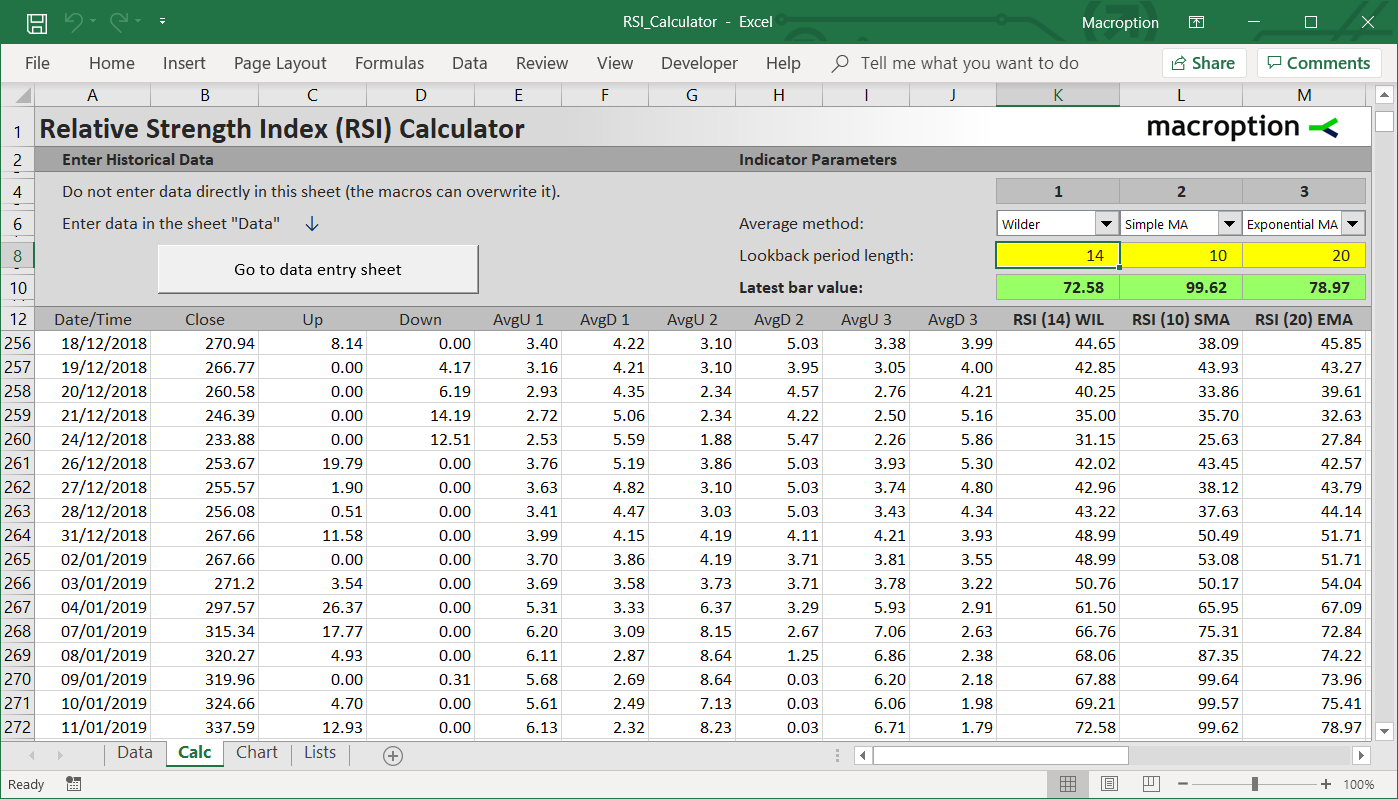• Intuitive navigation – you can start using it immediately
• User guide explaining all the calculation methods
• Works in all versions of Excel from Excel 97 to the latest, including Excel for Mac

\$39 one-time payment

You can find more details about individual features, exact contents of the user guide, and screenshots below:

## Supported RSI Calculation Methods

The calculator can calculate RSI using any of the three popular methods.

The methods differ in the exact formula for average up and down moves (the second step in RSI calculation).

The three methods are:

• Simple moving average
• Exponential moving average: a = 2/(n+1)
• Wilder's smoothing method: a = 1/n

The calculation methods are explained in chapter 4 of the user guide.

## RSI Period Length

You can set any period length on any of the calculation methods.

Note: The original RSI as introduced by J. Welles Wilder Jr. is Wilder's method with period length equal to 14.

## RSI Calculator User Guide

The user guide provides detailed instructions and notes for using the calculator. It also provides detailed explanation of RSI calculation, the differences between individual calculation methods, the effect of parameters, and interpretation of RSI values. You can find an overview of the contents below:

1. Introduction ...3
2. Structure and Basic Guidelines ...4
3. Entering Market Data ...5
4. Calculations and Parameters ...12
5. Charts ...19
6. Macro-Free Version ...21
7. Common Technical Problems ...23
8. Contact, Disclosures, and Disclaimer ...25

\$39 one-time payment

## Screenshots – This is How the Calculator Looks:

This is the main calculation sheet: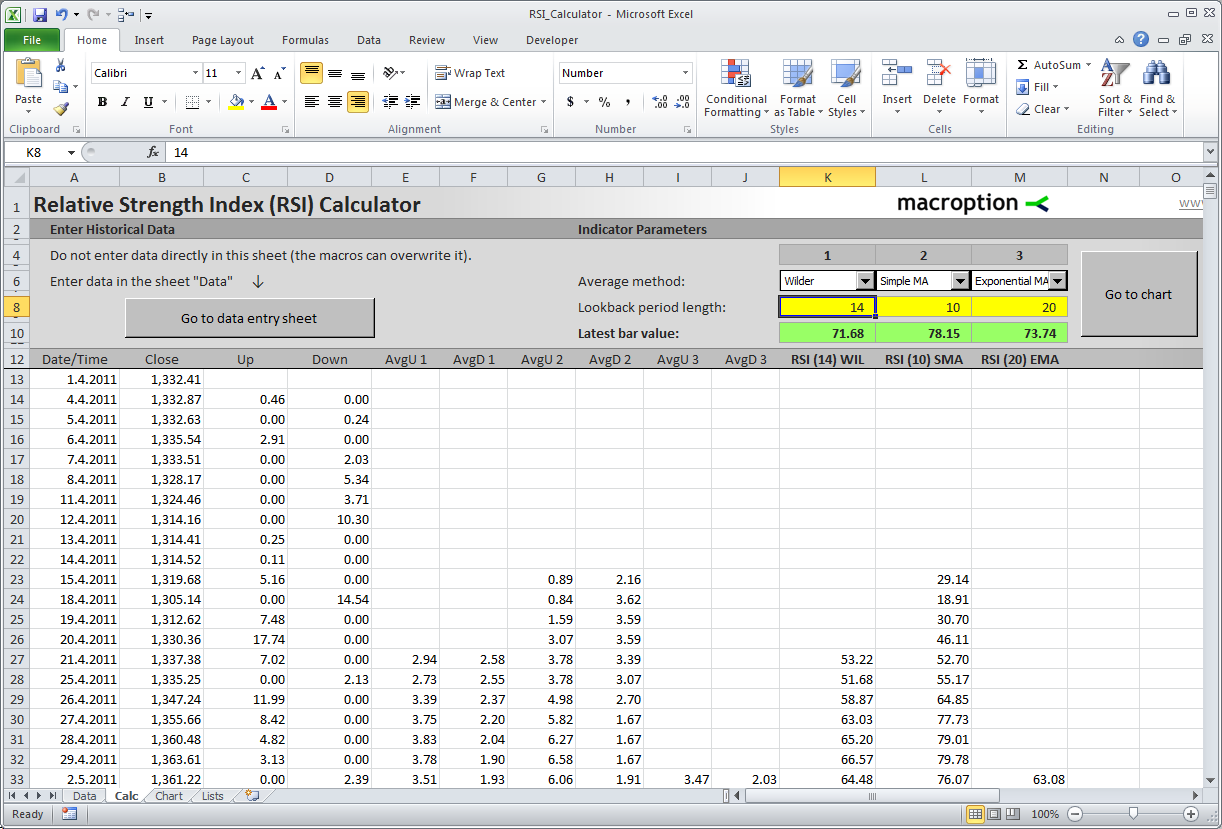You can calculate up to three different RSI indicators at the same time (in columns K-M).

Here you can set parameters for the indicators: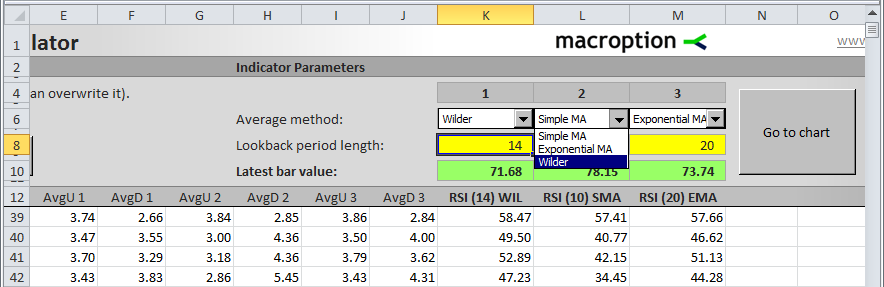You can choose from the three calculation methods (simple moving average, exponential moving average, and the original Wilder's smoothing). You can set any period length in the yellow cells (K8-M8).

The chart sheet displays 2 charts: the upper chart with price and the lower chart with the indicators.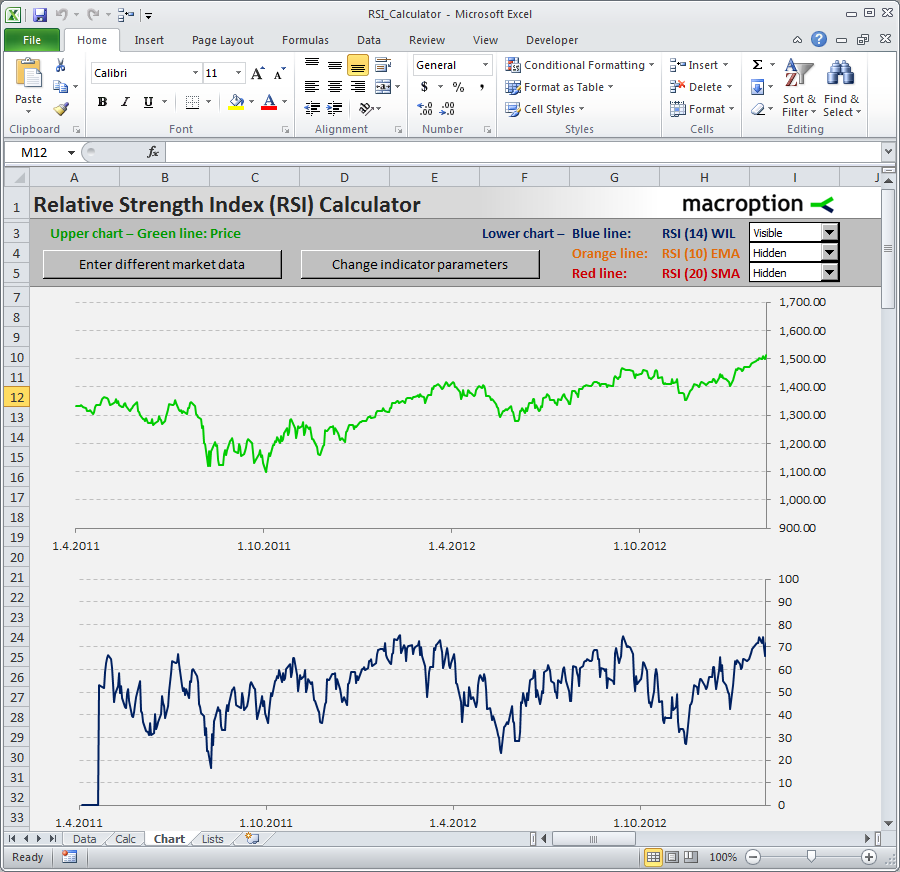Here you can set which of the indicators to display: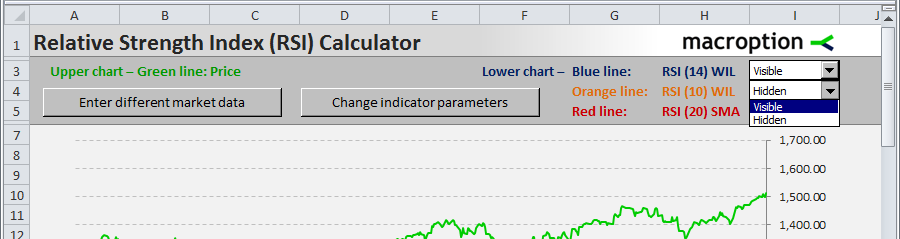Chart scales are adjusted automatically by a macro.

This is the sheet where you can enter data.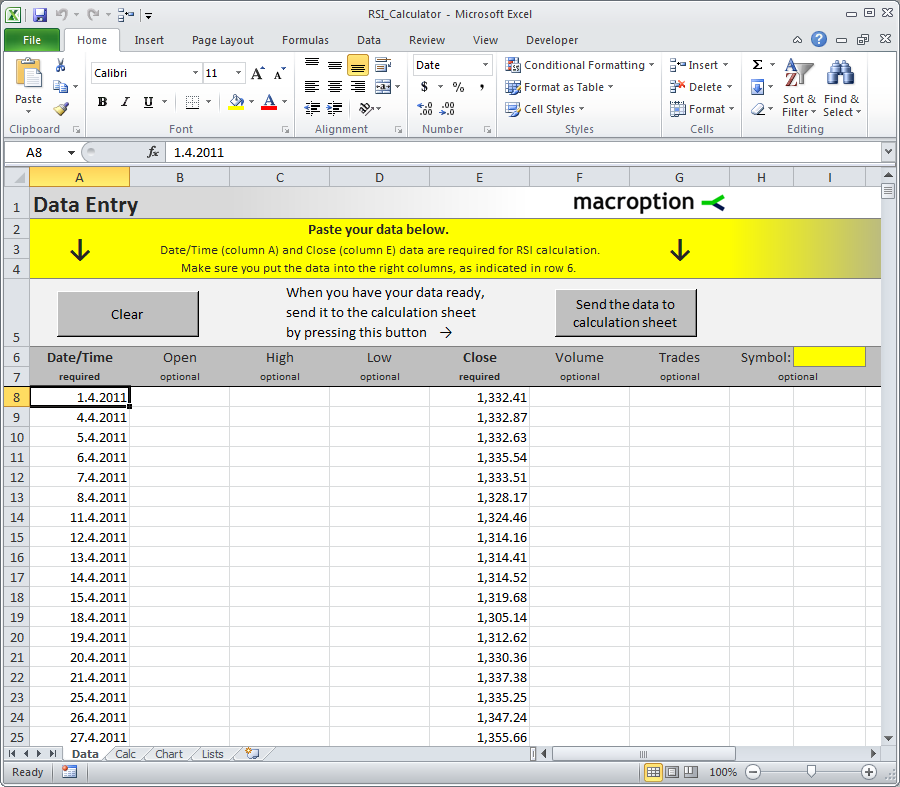Clicking the button on the right will sort the data from oldest to newest, import it into the calculation sheet, and build formulas. The macro will also check your data for errors, such as missing prices or non-numeric cells.

## Excel Versions and Macros

The default version of the calculator uses Excel macros to make it convenient and easy to use. If you can't or don't want to use macros, there is also a macro-free version available.

### All Excel Versions:

• RSI_Calculator.xlsm = the default version (all features, easiest to use)
• RSI_Macro-Free.xlsx = macro-free version (if you can't use macros)
• RSI_for_Excel_97-2003.xls = Excel 97-2003 version
• RSI_for_Excel_97-2003_Macro-Free.xls = macro-free Excel 97-2003 version

Every user gets access to all versions plus the user guide in pdf.

\$39 one-time payment